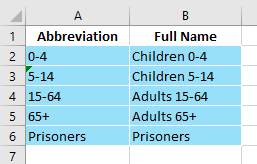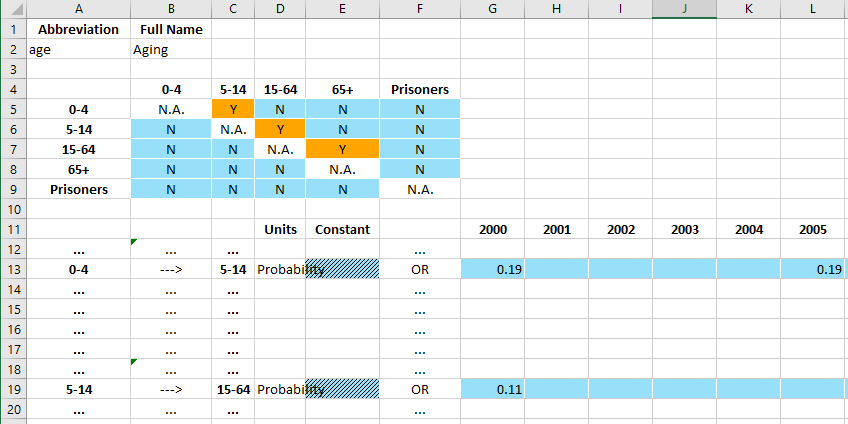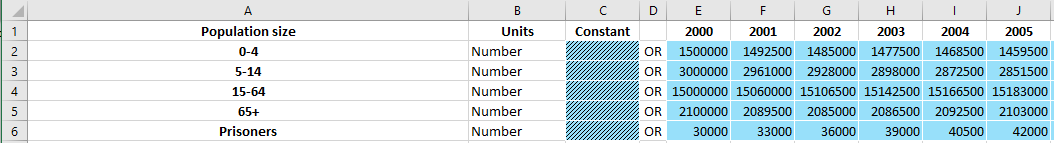# Data and Databooks¶

This page provides an overview of Atomica’s internal representation of databooks. Project data for an application is specified in a ‘databook’. This is an Excel file that contains

• A listing of which populations a simulation will have

• A specification of which transfers are present

• Population and time specific values for characteristics, parameters, transfers, and interactions

ProjectData is a Python object containing the data, and it has methods to convert to and from the databook spreadsheet.

:

import pandas as pd
import atomica as at
import matplotlib.pyplot as plt
import numpy as np

:

F = at.ProjectFramework(at.LIBRARY_PATH / "tb_framework.xlsx")

Parameter "lu_prog" is in rate units and a maximum value of "1" has been entered. Rates in the framework should generally not be limited to "1"
Parameter "lx_prog" is in rate units and a maximum value of "1" has been entered. Rates in the framework should generally not be limited to "1"
Parameter "leu_act" is in rate units and a maximum value of "1" has been entered. Rates in the framework should generally not be limited to "1"
Parameter "lex_act" is in rate units and a maximum value of "1" has been entered. Rates in the framework should generally not be limited to "1"
Parameter "llu_act" is in rate units and a maximum value of "1" has been entered. Rates in the framework should generally not be limited to "1"
Parameter "llx_act" is in rate units and a maximum value of "1" has been entered. Rates in the framework should generally not be limited to "1"
Parameter "l_inf" is in rate units and a maximum value of "1" has been entered. Rates in the framework should generally not be limited to "1"
Parameter "v_inf" is in rate units and a maximum value of "1" has been entered. Rates in the framework should generally not be limited to "1"
Parameter "lr_inf" is in rate units and a maximum value of "1" has been entered. Rates in the framework should generally not be limited to "1"
Parameter "ar_act" is in rate units and a maximum value of "1" has been entered. Rates in the framework should generally not be limited to "1"
Parameter "ar_rec" is in rate units and a maximum value of "1" has been entered. Rates in the framework should generally not be limited to "1"
Parameter "pd_rec" is in rate units and a maximum value of "1" has been entered. Rates in the framework should generally not be limited to "1"
Parameter "pm_rec" is in rate units and a maximum value of "1" has been entered. Rates in the framework should generally not be limited to "1"
Parameter "px_rec" is in rate units and a maximum value of "1" has been entered. Rates in the framework should generally not be limited to "1"
Parameter "nd_rec" is in rate units and a maximum value of "1" has been entered. Rates in the framework should generally not be limited to "1"
Parameter "nm_rec" is in rate units and a maximum value of "1" has been entered. Rates in the framework should generally not be limited to "1"
Parameter "nx_rec" is in rate units and a maximum value of "1" has been entered. Rates in the framework should generally not be limited to "1"
Parameter "pd_term" is in rate units and a maximum value of "1" has been entered. Rates in the framework should generally not be limited to "1"
Parameter "pm_term" is in rate units and a maximum value of "1" has been entered. Rates in the framework should generally not be limited to "1"
Parameter "px_term" is in rate units and a maximum value of "1" has been entered. Rates in the framework should generally not be limited to "1"
Parameter "nd_term" is in rate units and a maximum value of "1" has been entered. Rates in the framework should generally not be limited to "1"
Parameter "nm_term" is in rate units and a maximum value of "1" has been entered. Rates in the framework should generally not be limited to "1"
Parameter "nx_term" is in rate units and a maximum value of "1" has been entered. Rates in the framework should generally not be limited to "1"


The ProjectData object contains a number of attributes - pops is an odict storing populations and their full names. In the future, it may also store other attributes such as species - tvec stores the default time array associated with the data (users can override this on a per-table basis) - transfers and interactions store pairwise time series linking populations - tdve stores a dict of TimeDependentValueEntry tables for characteristics and parameters - tdve_pages stores a dict assigning TDVE tables to worksheets

There are three basic elements to the databook

1. The population table

2. TimeDependentConnection tables which are used for transfers and interactions. These have a ‘to’ and a ‘from’ population

3. TimeDependentValueEntry tables which are used for characteristics and parameters

## TimeSeries objects¶

Time-varying data is backed by the TimeSeries object defined in structure.py. This object stores sparse time-varying data, together with an assumption. This allows it to keep track of both assumption and time-varying values entered in a databook. It has a few key properties

:

ts = at.TimeSeries(t=[2014,2015],vals=[1,2])


A TimeSeries has t and vals attributes that store the time varying data

:

ts.t

:

[2014, 2015]

:

ts.vals

:

[1.0, 2.0]


The values can be modified using the insert and remove methods. These preserve the order of the times. Notice that the remove method removes data based on the time value rather than the index.

:

ts.insert(2016,3)
ts.remove(2015)
ts.vals

:

[1.0, 3.0]


A TimeSeries object also has an interpolate method to query its value at arbitrary times. It uses the same interpolation as for parameters, with constant extrapolation

:

t = np.arange(2013,2018,0.2)
plt.plot(t,ts.interpolate(t))

:

[<matplotlib.lines.Line2D at 0x7f0e5aec1750>]One of the special features of the TimeSeries object that caters for databook entry is the fact that it can independently store the constant assumption value. This is set by inserting a value with no time associated with it

:

ts2 = at.TimeSeries()
ts2.insert(None,2)
ts2.assumption

:

2.0


Since the data for a TimeSeries could be in assumption or in vals, the method get_arrays allows you to get time and value arrays for the content of the TimeSeries

:

t,v = ts.get_arrays()
print(t)
print(v)

t,v = ts2.get_arrays()
print(t)
print(v)

[2014 2016]
[1. 3.]
[nan]
[2.]


However, the most common usage for the TimeSeries is to turn sparse data into full interpolated arrays. The interpolate method automatically manages the assumption and the time dependent values, so that you don’t have to do this yourself:

:

t = np.arange(2013,2018,0.2)
plt.plot(t,ts.interpolate(t),label='Time varying')
plt.plot(t,ts2.interpolate(t),label='Assumption')
plt.legend()

:

<matplotlib.legend.Legend at 0x7f0e5ad08e90>The TimeSeries object also has a units property that lets it store the units of the quantity entered in the databook.

In general, whenever the databook has a time-dependent values row with an assumption and some year-specific data, the corresponding object used to store the data on that row is a TimeSeries

### Population table¶

The population table is very basic:in the future, there will probably be more columns - for example, to separate human and mosquito populations (these would need to be validated against tags specified in the Framework)

### TimeDependentConnections¶A TimeDependentConnections table consists of three elements

1. A code name/full name table at the top

2. A matrix of Y/N values that controls which time series appear on the page

3. A set of time series inputs where the user enters time-varying data

This table is managed by the TimeDependentConnections object in excel.py which has

• A code name

• A full name

• The type (whether it’s an interaction or a transfer - the difference being, a transfer cannot have any entries on the diagonal i.e. within the same population)

• A list of population names

• A list of times

• A dict of TimeSeries objects keyed by the (to,from) populaton names

• A method from_tables() to construct a TimeDependentConnections instance based on the rows in the spreadsheet

• A method write() that takes in a sheet and a start row, and writes the content for the object to the spreadsheet

### TimeDependentValuesEntry¶A TimeDependentValuesEntry consists of a single element with

• The full name of the quantity at the top left

• The left column with population names

• Value entry cells for contant and year-specific values

A code name/full name table at the top A matrix of Y/N values that controls which time series appear on the page A set of time series inputs where the user enters time-varying data

This table is managed by the TimeDependentValuesEntry object in excel.py which has

• A name

• The time vector

• A dict of TimeSeries objects keyed by the population name

• A method from_rows() to construct a TimeDependentValuesEntry instance based on the rows in the spreadsheet

• A method write() that takes in a sheet and a start row, and writes the content for the object to the spreadsheet

## ProjectData operations¶

A number of possible operations can be performed on ProjectData

• Get a new instance using ProjectData.new() where you pass in a Framework together with a list (or number) or populations and transfers

• Use to_spreadsheet() to get a sciris Spreadsheet representation of the data, and save() to write the ProjectData to disk

• Use get_ts() to get the time-data associated with a quantity out of ProjectData e.g. when making parameters

• You can add or remove populations and transfers while keeping data intact

• You can change the time values using change_tvec() while keeping the data intact

Note that you can also change the time values in the spreadsheet in place just by adding or removing columns. In principle, you can also specify values only for a subset of populations, but at the moment this is probably not propagated through the model yet.